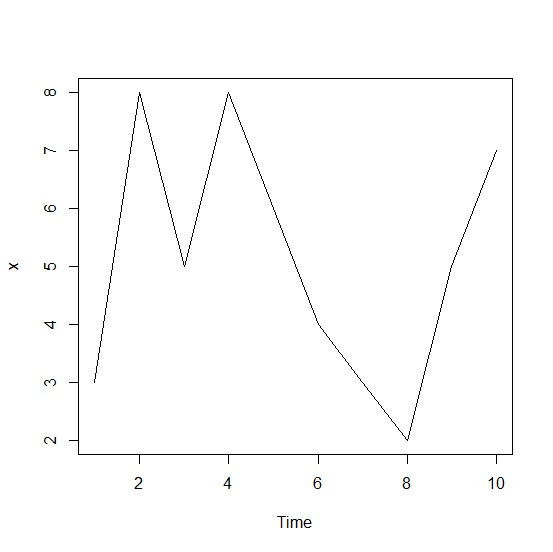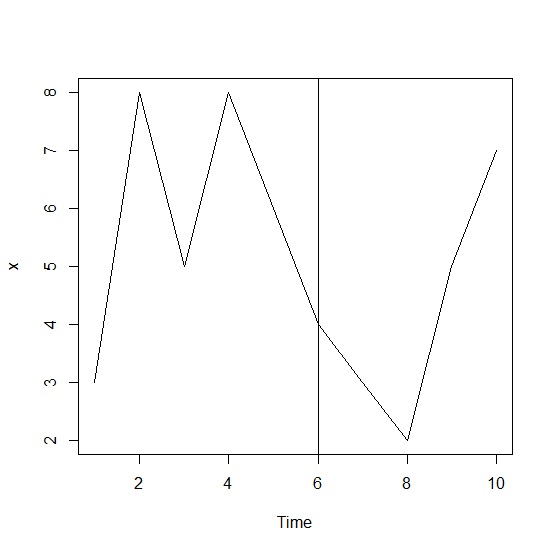# How to create a vertical line in a time series plot in base R?n

To create a time series plot, we can use simply apply plot function on time series object and if we want to create a vertical line on that plot then abline function will be used with v argument.

For example, if we have a time series object called T and we want to create a time series plot of T with vertical line at point 5 then we can use the below given command after creating the plot −

abline(v=5)

## Example

To create a vertical line in a time series plot in base R, use the following code −

x<-ts(rpois(10,5))
x
Time Series:
Start = 1
End = 10
Frequency = 1

If you execute the above given code, it generates the following output −

 3 8 5 8 6 4 3 2 5 7


To create a vertical line in a time series plot in base R, add the following code to the above code −

x<-ts(rpois(10,5))
plot(x)

## Output

If you execute all the above given codes as a single program, it generates the following output −To create a vertical line in a time series plot in base R, add the following code to the above code −

x<-ts(rpois(10,5))
plot(x)
abline(v=6)

## Output

If you execute all the above given codes as a single program, it generates the following output −